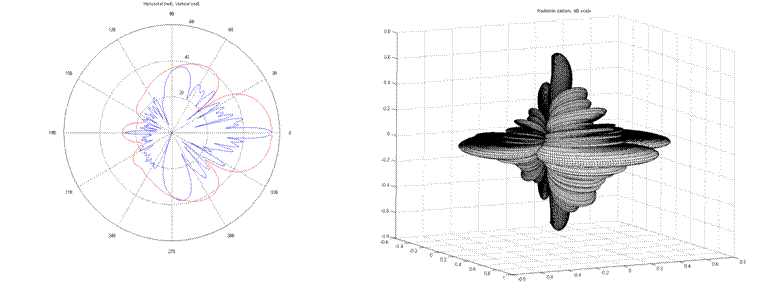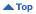Sunday, May 19 2019 12:47 UT

# VHF and UHF Area Prediction Tool Details

## Digital Elevation Models and Raster Imagery

• Main input data source being DEM (Digital Elevation Models) derived from Satellite and LIDAR sources.
• DEMs measure the highest point below a nominal observer hovering the earth (data can include buildings and trees).
• Imported into software in square tile or irregular format.
• Variable resolution from 5m to 1km.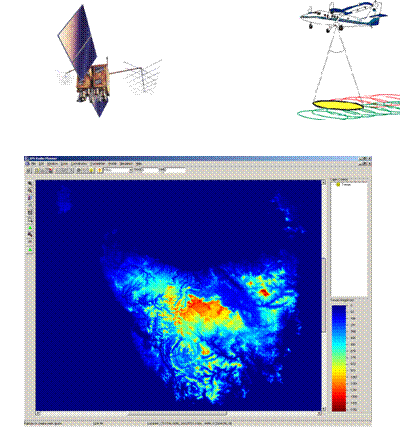## Mapping and Coordinate Systems

• GIS requirements for use on Australian terrain data.
• Incorporating Australian AGD66, AGD84 and GDA94 datums (GRS80 ellipsoid) and equivalent UTM projections for grid coordinates AMG66, AMG84 and MGA94.
• Operations to perform conversions between Grid coordinates (Eastings/Northings) and Geographical (Latitude/Longitude) using Redfearn's formulae.
• Distance and Height Scale factors for accurate distance calculations on the ellipsoid.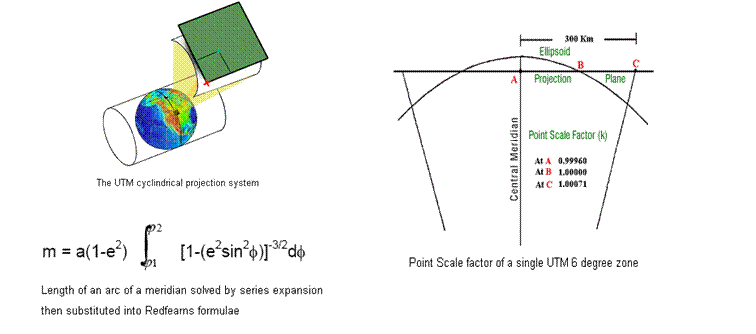## Empirical Propagation Models

• ITU recommended Empirical Pathloss models such as Okumura-Hata and Longely-Rice
• Okumura-Hata model variations for Large Cities, Medium Cities, Suburban Area and Open/Rural Areas. Valid for:
• 150MHz < f < 1500MHz
• 30m < Htx < 200m
• 1m < Hrx < 10m
• 1km < d < 20km
• COST 231 Hata model for 1500MHz-2000MHz.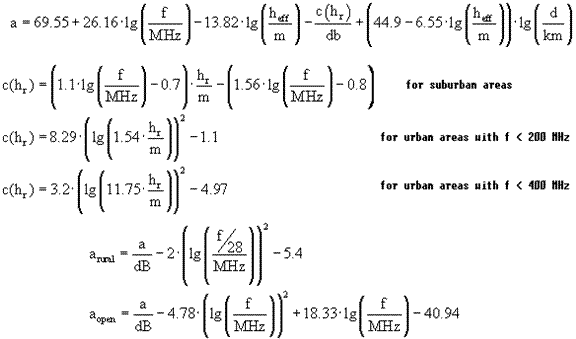## Knife Edge Diffraction

• Semi Deterministic pathloss models employ knife edge diffraction for evaluating hilly terrain and finding losses in shadowed regions.
• A terrain cross section profile is produced between the Tx and Rx which is then passed through a convex hull function to find diffracting radio path.
• Decision calculations based on the knife edge model are performed to produce the Fresnel-Kirchoff diffraction parameter ν.
• Fresnel-Kirchoff parameter then substituted into Lee's approximation of attenuation over single diffracting edge.
• Used in conjunction with the Friis transmission equation for pathloss (dependent upon Fresnel zone clearance).

Path loss (dB) = 32.44 + 20 log d (km) + 20 log f (MHz)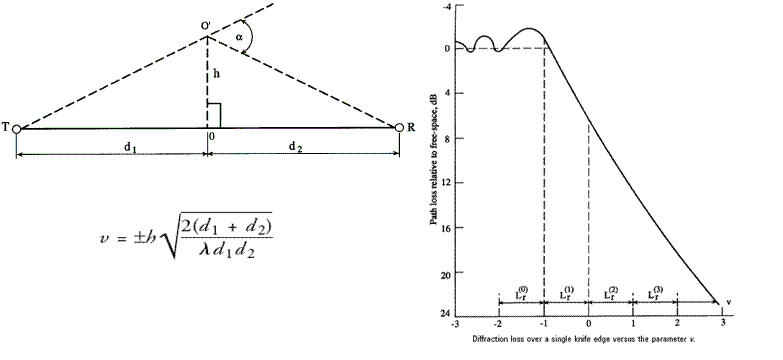Each model differs in its approach to determining the inputs to the Fresnel-Kirchoff diffraction parameter ν equation, and for what edge contributes most to the loss.

• Bullington model below takes simplest, least accurate approach and reduces the profile to a single knife edge.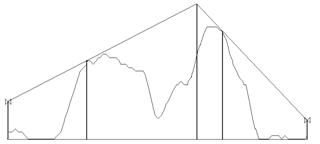• Epstein-Peterson model below considers each significant knife edge individually and sums each loss over the diffracting path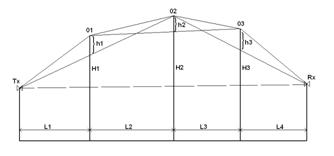• Giovanelli method below identifies a dominant edge and calculates each loss with respect to it, but creates separate observation planes for each edge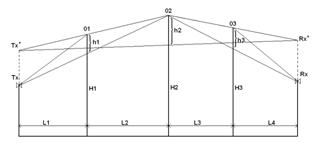• Deygout model below identifies a dominant knife edge and calculates all losses with respect to it.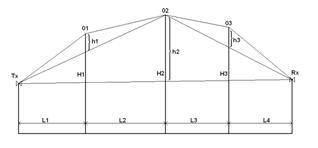## Antenna modeling

• Vertical and Horizontal gain patterns loaded in from a manufacturers antenna data file.
• Pattern multiplication performed for an approximate 3D representation.

• Full gain pattern can be incorporated into propagation model via a simple ray-trace function and added to the pathloss equation.